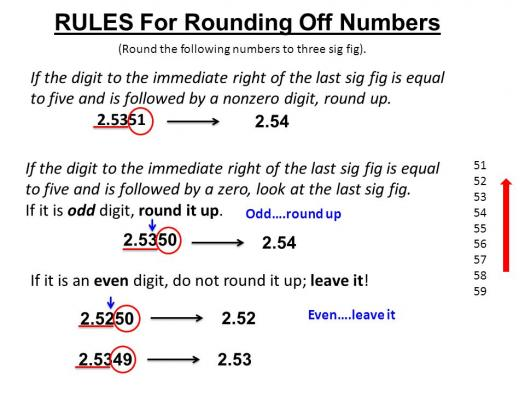10 Questions | Total Attempts: 389SettingsWe round off numbers all the time, and we've all learned to do so effectively at school before we've actually used this technique in our everyday lives. But, do you actually know the rules that make up this technique? Do you think you can pass our quiz this time? Take our quiz and find out.

• 1.
What's the purpose of rounding a number?
• A.

When pressed with time

• B.

To read it with more ease

• C.

To report and communicate a value that is easier than the original one

• D.

To help those who are not very good at calculating values

• 2.
What's a fixed-point number?
• A.

It is a point of the curve of values

• B.

It is a real data type for a number that has a variable number of digits after the redix point

• C.

It is a real data type for a number that has a fixed number of digits after the redix point

• D.

It is a point equal to 0

• 3.
What are significant figures?
• A.

They are digits that carry meaning contributing to its measurement resolution

• B.

They are figures greater than 100

• C.

They are figures greater than 10

• D.

They are figures with a great resolution

• 4.
What's a digital signal?
• A.

It's a signal only measured via the internet

• B.

It is a discrete-time signal for which not only the time but also the amplitude has discrete values

• C.

It's a signal only measurable via satellite

• D.

It's a signal with 2 digits

• 5.
What's a rational number?
• A.

Any number that can be expressed as the quotient or fraction p/q of two integers

• B.

Any number that can be expressed as the quotient or fraction

• C.

A real number

• D.

A number found between 1 and 9

• 6.
What's the other expression for rounding down?
• A.

Finding a whole number

• B.

Finding a smaller value

• C.

Going down

• D.

Taking the floor

• 7.
What's the other expression for rounding up?
• A.

Taking the ceiling

• B.

Finding a greater number

• C.

Finding a number over 100

• D.

Taking the floor

• 8.
What's the other expression for rounding towards zero?
• A.

To subtract

• B.

To cut

• C.

To truncate

• D.

To divide

• 9.
• A.

24

• B.

23

• C.

23.4

• D.

23.55

• 10.
• A.

-24

• B.

-22

• C.

-23

• D.

-24.5

Related TopicsBack to top